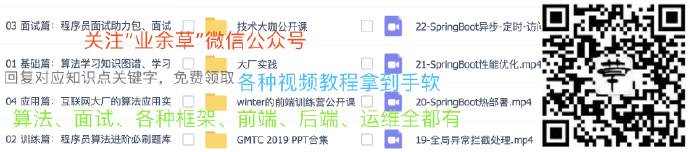# MyBatis-Plus中的QueryWrapper、UpdateWrapper用法教程

JAVA 5253浏览## eq 的用法：

``````eq(R column, Object val)
eq(boolean condition, R column, Object val)
// 例: eq("name", "业余草")--->name = '业余草'``````

## ne 函数的用法：

``````ne(R column, Object val)
ne(boolean condition, R column, Object val)
// 不等于 <>
// 例: ne("name", "业余草")--->name <> '业余草'``````

## gt 函数的用法：

``````gt(R column, Object val)
gt(boolean condition, R column, Object val)
// 大于 >
// 例: gt("age", 18)--->age > 18``````

## ge 函数的用法：

``````ge(R column, Object val)
ge(boolean condition, R column, Object val)
// 大于等于 >=
// 例: ge("age", 18)--->age >= 18``````

## lt 函数的用法：

``````lt(R column, Object val)
lt(boolean condition, R column, Object val)
// 小于 <
// 例: lt("age", 18)--->age < 18``````

## le 函数的用法：

``````le(R column, Object val)
le(boolean condition, R column, Object val)
// 小于等于 <=
// 例: le("age", 18)--->age <= 18``````

## between 函数的用法：

``````between(R column, Object val1, Object val2)
between(boolean condition, R column, Object val1, Object val2)
// BETWEEN 值1 AND 值2
// 例: between("age", 18, 30)--->age between 18 and 30``````

## notBetween 函数的用法：

``````notBetween(R column, Object val1, Object val2)
notBetween(boolean condition, R column, Object val1, Object val2)
// NOT BETWEEN 值1 AND 值2
// 例: notBetween("age", 18, 30)--->age not between 18 and 30``````

## like 函数的用法：

``````like(R column, Object val)
like(boolean condition, R column, Object val)
// LIKE '%值%'
// 例: like("name", "王")--->name like '%王%'``````

## notLike 函数的用法：

``````notLike(R column, Object val)
notLike(boolean condition, R column, Object val)
// NOT LIKE '%值%'
// 例: notLike("name", "王")--->name not like '%王%'``````

## likeLeft 函数的用法：

``````likeLeft(R column, Object val)
likeLeft(boolean condition, R column, Object val)
// LIKE '%值'
// 例: likeLeft("name", "王")--->name like '%王'``````

## likeRight 函数的用法：

``````likeRight(R column, Object val)
likeRight(boolean condition, R column, Object val)
// LIKE '值%'
// 例: likeRight("name", "王")--->name like '王%'``````

## isNull 函数的用法：

``````isNull(R column)
isNull(boolean condition, R column)
// 字段 IS NULL
// 例: isNull("name")--->name is null``````

## isNotNull 函数的用法：

``````isNotNull(R column)
isNotNull(boolean condition, R column)
// 字段 IS NOT NULL
// 例: isNotNull("name")--->name is not null``````

## in 函数的用法：

``````in(R column, Collection value)
in(boolean condition, R column, Collection value)
// 字段 IN (value.get(0), value.get(1), …)
// 例: in("age",{1,2,3})--->age in (1,2,3)

in(R column, Object… values)
in(boolean condition, R column, Object… values)
// 字段 IN (v0, v1, …)
// 例: in("age", 1, 2, 3)--->age in (1,2,3)``````

## notIn 函数的用法：

``````notIn(R column, Collection value)
notIn(boolean condition, R column, Collection value)
// 字段 NOT IN (value.get(0), value.get(1), …)
// 例: notIn("age",{1,2,3})--->age not in (1,2,3)

notIn(R column, Object… values)
notIn(boolean condition, R column, Object… values)
// 字段 NOT IN (v0, v1, …)
// 例: notIn("age", 1, 2, 3)--->age not in (1,2,3)``````

## 各 wapper 介绍 ：

• Wrapper ： 条件构造抽象类，最顶端父类，抽象类中提供4个方法西面贴源码展示
• AbstractWrapper ： 用于查询条件封装，生成 sql 的 where 条件
• AbstractLambdaWrapper ： Lambda 语法使用 Wrapper统一处理解析 lambda 获取 column。
• LambdaQueryWrapper ：看名称也能明白就是用于Lambda语法使用的查询Wrapper
• LambdaUpdateWrapper ： Lambda 更新封装Wrapper
• QueryWrapper ： Entity 对象封装操作类，不是用lambda语法
• UpdateWrapper ： Update 条件封装，用于Entity对象更新操作

## 参考资料